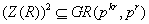1. ### Additive Functions from Measure Theory Point-View

Abstract: We consider the behavior of additive functions from the measurability point of view. Some related questions concerning measurability properties of additive functions with respect to various classes of measures are also discussed.

Keywords: additive function, measurability of function, thick graph.

2. ### On the Zero Divisor Graphs of Finite Rings in Which the Product of Any Two Zero Divisors Lies in the Coefficient Subring

Abstract: Let r be a positive integer and 2≤k∈Z. Let GR(pkr, pk) be a Galois ring of order pkr and characteristic pk. Consider, R = GR(pkr, pk) ⊕U where U is a finitely generated GR(pkr, pk) module. If Z(R) is the set of zero divisors in R satisfying the conditionthen it is well known that R is a completely primary finite ring and the structure of its group of units has been studied before. In this paper, we study the structure of its zero divisors via the zero divisor graphs.

Keywords: Finite rings, Zero divisor graphs.

3. ### A Robust Procedure for the Fit of Oneway ANOVA Model under Adaptation on the Observed Samples

Afrifa-Yamoah, E., Okyere, Gabriel Asare, Asare-Bediako, Michael, Bashiru I.I. Saeed

Abstract: The possible dominance of basic assumption about underlying models on the analysis of data is of much concern. This study aimed develop a robust fitting procedure for one-way ANOVA models under adaption on the observed samples. Further investigation on Asymptotic Relative Efficiency (ARE) of this procedure and parametric F-test under class of continuous distributions was performed. 10,000 simulations were carried out for a one-way ANOVA model with three levels for sample sizes 5, 10, 15, and 20. Intralevel correlation coefficient ρ = 0 was considered in the these simulations. The findings revealed that the parametric F-test for oneway ANOVA model performed better than the non-parametric Adaptive test proposed for symmetric and moderate tailed distributions and then in symmetric and light tailed distributions with ARE between 2% and 55%. However, the Adaptive test outperformed the F-test in symmetric and asymmetric with varying tail weights distributions with ARE between 4% and 64%. Although, the F-test displayed superiority in efficiency in symmetric medium and light tailed distributions, the Adaptive test was more efficient in more broader class of continuous distribution.

Keywords: adaptive, selector statistic, simulation, non-parametric, continuous distribution, Asymptotic Relative Efficiency (ARE).

4. ### Feedback Effects Based on an Interregional Input – Output Model for Greece

Erasmia Valma

Abstract:

The main objective of this paper is centered to analyze the interregional linkages in order to measure the feedback effects.

The analysis will be implemented with a two region interregional model for the Greek economy constructed by the author by own forces.

Feedback analysis offers additional information in calculating output multipliers. The feedback effects were introduced as the increase in outputs originating in one region that has the possibility to influence production levels in another region and will affect activity back in the first region of origin.

The results of how are spread widely the effects in the economy is important to identity production and establish targets for regional growth.

Keywords: Interregional model, feedback effects, output multipliers, interregional output multipliers.

Laczka Éva